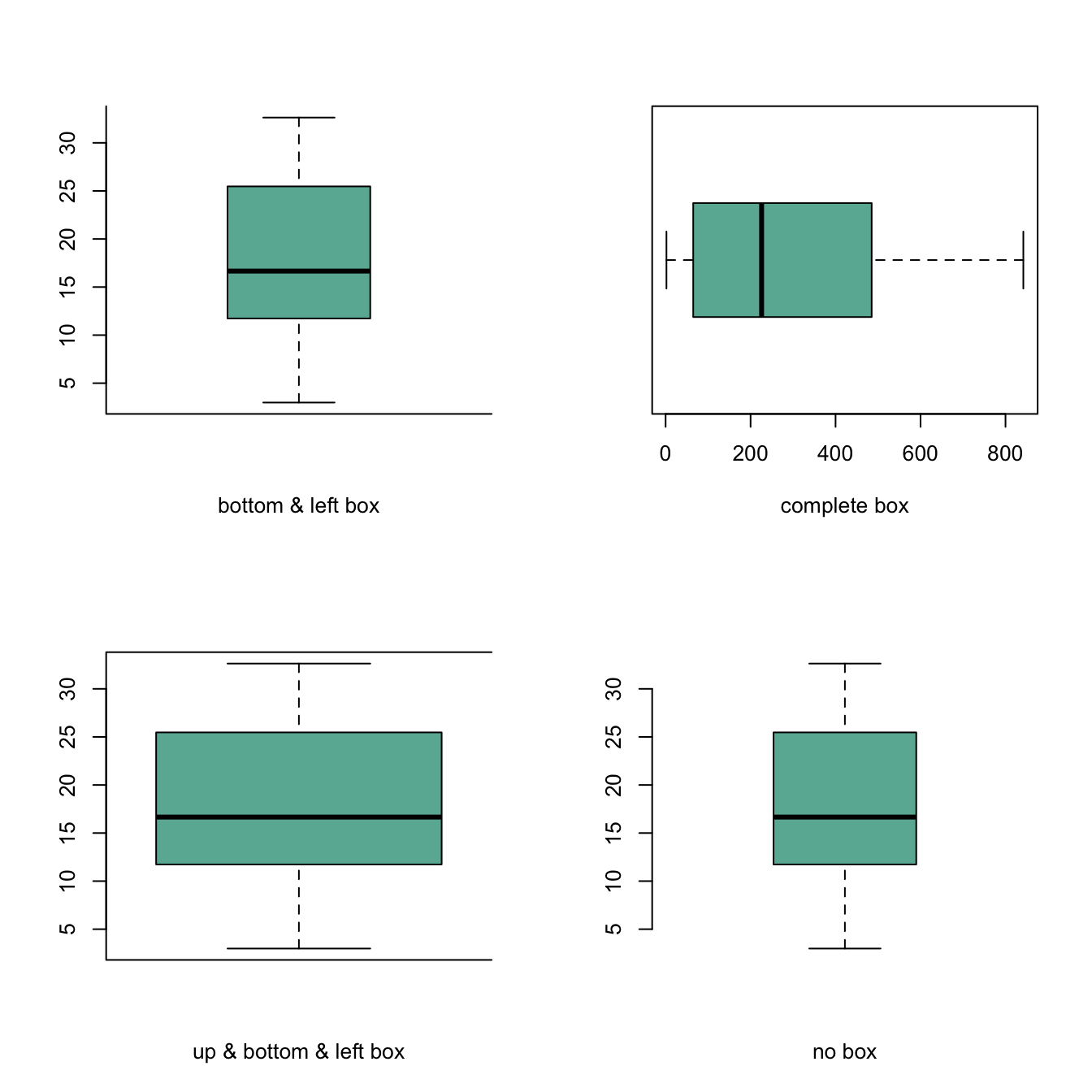# Control box type with the bty option

The `bty` option of the `par()` function allows to control the box style of base R charts. This post provides a few examples illustrating how this option works.

The `bty` option of the `par()` function allows to custom the box around the plot.

Several letters are possible. Shape of the letter represents the boundaries:

• `o`: complete box (default parameter),
• `n`: no box
• `7`: top + right
• `L`: bottom + left
• `C`: top + left + bottom
• `U`: left + bottom + right``````# Cut the screen in 4 parts
par(mfrow=c(2,2))

#Create data
a=seq(1,29)+4*runif(29,0.4)
b=seq(1,29)^2+runif(29,0.98)

# First graph
par(bty="l")
boxplot(a , col="#69b3a2" , xlab="bottom & left box")
# Second
par(bty="o")
boxplot(b , col="#69b3a2" , xlab="complete box", horizontal=TRUE)
# Third
par(bty="c")
boxplot(a , col="#69b3a2" , xlab="up & bottom & left box", width=0.5)
# Fourth
par(bty="n")
boxplot(a , col="#69b3a2" , xlab="no box")``````

Related chart types

## Contact

This document is a work by Yan Holtz. Any feedback is highly encouraged. You can fill an issue on Github, drop me a message on Twitter, or send an email pasting yan.holtz.data with gmail.com.